## LetsPlayMaths.Com

WELCOME TO THE WORLD OF MATHEMATICS

# Class 2 Money

Money

Conversion of Rupees into Paise

Conversion of Paise into Rupees

Addition of Money

Subtraction of Money

Money Test

Money Worksheets

Answer Sheet

## Money

In India following coins are used.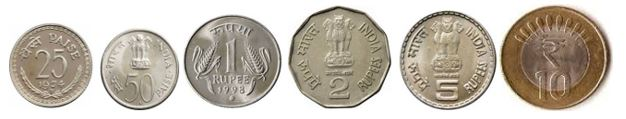Following currency notes are used in our country.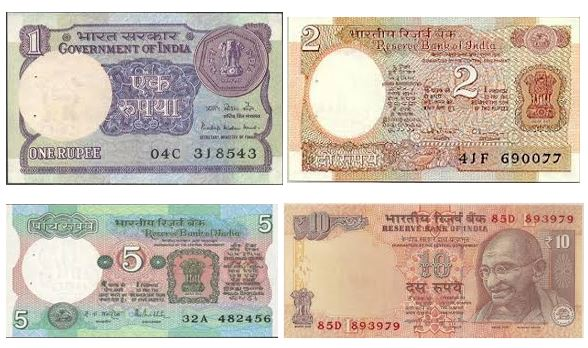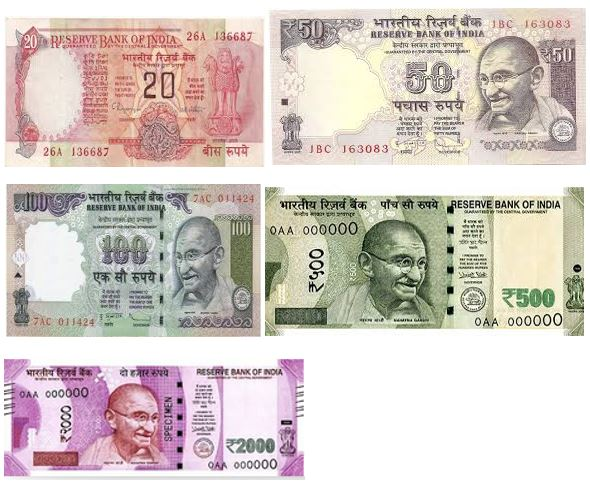Suppose you have 20 rupee note and 50 paisa coin. In words we express this amount as twenty rupees
and fifty paisa.In figures we write it as ₹ 20.50.

₹ - International symbol for Rupees
25 rupees we write as Rs. 25 or ₹ 25
When we write rupees and paise together, for example 25 rupees and 75 paise, we write Rs. 25.75.
Rupees and paise are separated by a dot.

## Conversion of Rupees into Paise

To convert rupees into paise, we must multiply the amount in rupees by 100. Let’s see some examples.

Example 1. Convert ₹ 5 into paise.

Solution. ₹ 5 = 5 X 100 = 500 p

Example 2. Convert ₹ 15.75 into paise.

Solution. ₹ 15.75 = 15.75 X 100 = 1575 p

## Conversion of Paise into Rupees

To convert paise into rupees, we place a dot after two digits from right of the number. Number on the left of the dot denotes number of rupees. Number on the right of the dot denotes paise. Let’s see some examples.

Example 1. Convert 900 paise into rupees.

Solution. 900 p = ₹ 9.00

Example 2. Convert 1225 paise into rupees.

Solution. 1225 p = ₹ 12.25 (12 rupees and 25 paise)

## Addition of Money

Addition of money is like normal addition, here we must add the Paise first and then add the rupees.
Have a look at the given examples.

Example 1. Add Rs. 25.20 and Rs 15.25

Solution.Arrange the numbers in tabular format as shown below.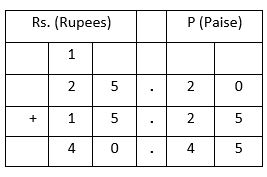Add the paise  : 20p + 25p = 45p

Add the rupees : ₹ 25 + ₹ 15 = ₹ 40

So, the result is ₹ 40.45

Example 2. Add ₹ 55.50 and ₹ 24.75

Solution.Arrange the numbers in tabular format as shown below.Add the paise : 50p + 75p = 125p = 100p + 25p = ₹ 1 + 25p

₹ 1 carry over to rupees column and 25p remain in paise column.

Add the rupees : ₹ 55 + ₹ 24 + ₹ 1(Carry over) = ₹ 80

Write ₹ 80 in rupees column.

So, the result is ₹ 80.25.

## Subtraction of Money

Subtraction of money is like normal subtraction, here we must subtract the Paise first and then subtract the rupees.
Have a look at the given examples.

Example 1. Subtract ₹ 34.23 from ₹ 87.56

Solution.Arrange the numbers in tabular format as shown below.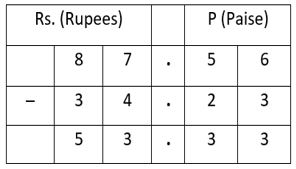Subtract the paise first

56p – 23p = 33p

Then subtract the rupees

₹ 87 – ₹ 34 = ₹ 53

So, the result is ₹ 53.33

Example 2. Subtract ₹ 26.75 from ₹ 58.

Solution.Arrange the numbers in tabular format as shown below.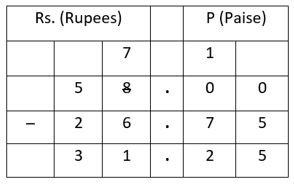Subtract the paise first. 75p can not be subtracted from 0 paise, that’s why we must borrow ₹ 1.

₹ 57 is remained in rupees column.

100p – 75p = 25p

Then subtract the rupees. ₹ 57 – ₹ 26 = ₹ 31

So, the answer is ₹ 31.25

Money Test - 1

Money Test - 2

Money Test - 3

Money Test - 4

## Class-2 Money Worksheet

Money Worksheet - 1

Money Worksheet - 2

Money Worksheet - 3

Money Worksheet - 4

Money Worksheet - 5

## Answer Sheet

Money-AnswerDownload the pdf

Copyright © 2021 LetsPlayMaths.com. All Rights Reserved.